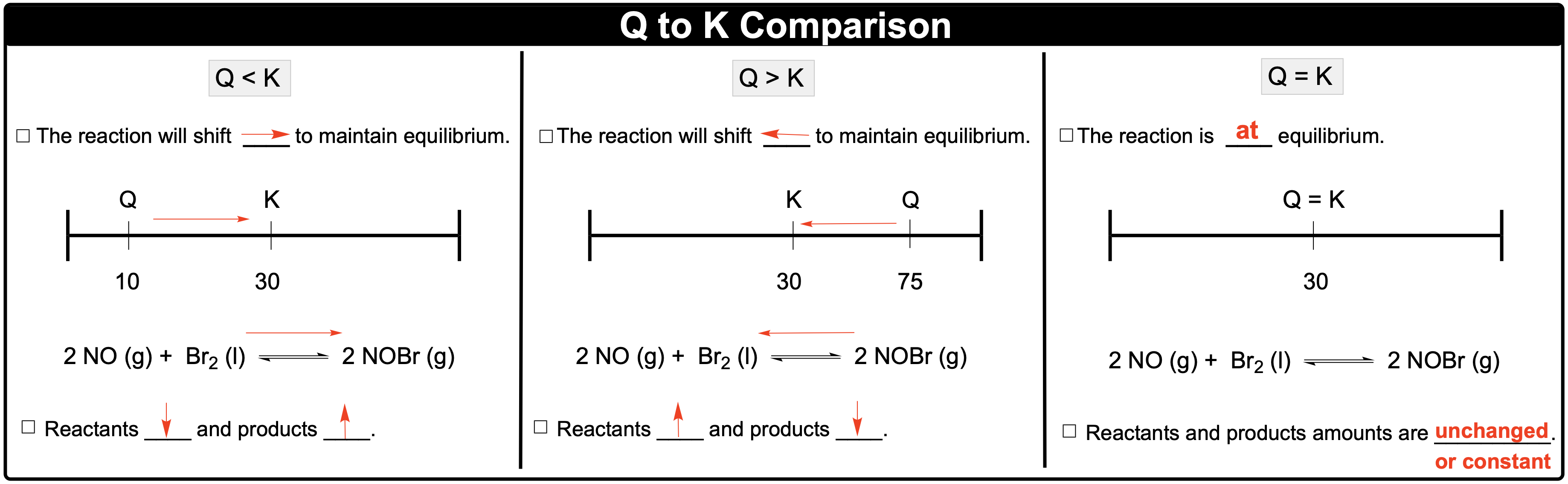Clutch Prep is now a part of Pearson
Ch.14 - Chemical EquilibriumWorksheetSee all chapters

# Reaction Quotient

See all sections
Sections
Intro to Chemical Equilibrium
Equilibrium Constant (K)
Equilibrium Constant Calculations
Kp and Kc
Using Hess's Law To Determine K
Calculating K For Overall Reaction
Le Chatelier's Principle
ICE Charts
Reaction Quotient

Reaction Quotient is a ratio of product to reactant concentrations and can be used to determine if a chemical reaction is at equilibrium or not.

###### Equilibrium Determination

Concept #1: Calculating the Reaction Quotient

Example #1: The formation of gaseous ammonia is displayed by the equation given below:

N2 (g) + H2 (g) ⇌ 2 NH3 (g)

What is the reaction quotient if the following amounts (in moles) of each component is placed in a 10.0 L vessel.

N2 = 0.650 moles                       H2 = 0.330 moles                       NH3 = 0.529 moles

Concept #2: Comparing Q to KComparing Q to K will determine the direction of the chemical shift.

Example #2: For the reaction: 4 HBr (g) + O2 (g)  ⇌ 2 Br2 (g) + 2 H­2O (l), the equilibrium constant is 0.063 at 400 K. If the reaction quotient is 0.100, which of the following statements is true?

a) [HBr] will decrease                 b) [O2] will increase                    c) [Br2] will increase                   d) [H2O] will increase

Practice: For the reaction: 2 CO2 (g)  ⇌ 2 CO (g) + 2 O2  (g), the equilibrium constant is 3.12 × 104 at 400 K, while the reaction quotient is 4.18 × 104. If initially we have 0.20 atm CO2, 0.30 atm CO and 0.15 atm O2, which of the following statements is not true?

a) The pressure of CO2 will be greater than 0.20 atm.

b) The pressure of CO will be less than 0.30 atm.

c) The pressure of O2 will be greater than 0.15 atm.

d) The pressure of O2 will be less than 0.15 atm.

e) The reaction will favor reactants.

Practice: The equilibrium constant for the following gas phase reaction is 0.75 at 750 K. After a short time, analysis of a small amount of the reaction mixture shows the concentrations to be [NOBr] = 1.25 M, [NO] = 0.80 M and [Br2] = 0.50 M. Which of the following statements is/are true?

2 NOBr (g)  ⇌ NO (g) + Br2 (g)

a) The reaction mixture is at equilibrium.

b) No further reaction will occur.

c) The partial pressure of NOBr will increase.

d) The partial pressure of NO will increase.

e) The reaction will shift to the left, the reactant side.